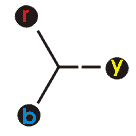# Power transformer:

The power transformer used to transfer the high voltage supply from one place to another. the power transformer mostly used in power generating station and bulk industry areas. for requiring more power in industrial areas they are using parallel connection of power transformer. the parallel connection of transformer required vector group test for combine two power transformer. here we will deatily discuss about vector group test of transformer.

## Vector Group Test of Transformer

The vector group transformer test is important for connecting two power transformer in parallel. that’s why every power transformer while manufacturing time they are done the vector group test. the vector group test used to identify the phase sequence of the transformer and also identify the which phase getting higher positive voltage. its also protect the short circuit between the phases.

for example we will consider two power transformer we are going to connect in parallel. both the transformer is having same phase sequence, ratings and both transformer having same number of primary turns and same number of secondary turns. the first transformer is connected in star connection and second transformer is connected in delta connection. if the same input voltage is applied to both primary side of the transformer. then secondary side of the both transformer is producing emf in each phase with respect to their primary side.

generally we know for star connection, the line voltage is equal to √3 times phase voltage and delta connection the line voltage is equal to phase voltage. here we connected first transformer secondary side in star connection, so the secondary voltage is √3 times of phase voltage of the secondary coil. but second transformer has connected in delta connection, so the secondary side line voltage is equal to phase voltage of the secondary coil. if you apply the vector diagram for these two transformer we will get 30o

The below table will help you to identify the vector group of the power transformer. and we can easily connecting the transformer in parallel connection.

GROUP Connection Connection
0
(0o)
Yy0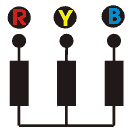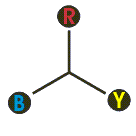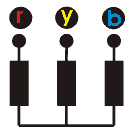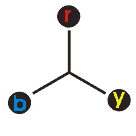Dd0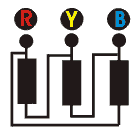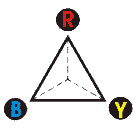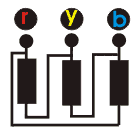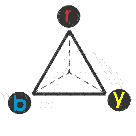1
( 30o)
Yd1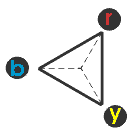Dy1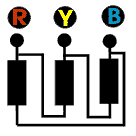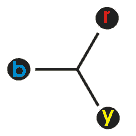6
( 180o)
Yy6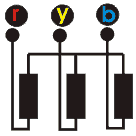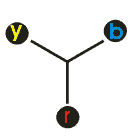Dd6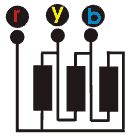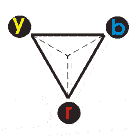11
( – 30o)
Yd11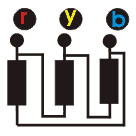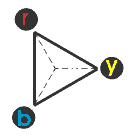Dy11